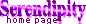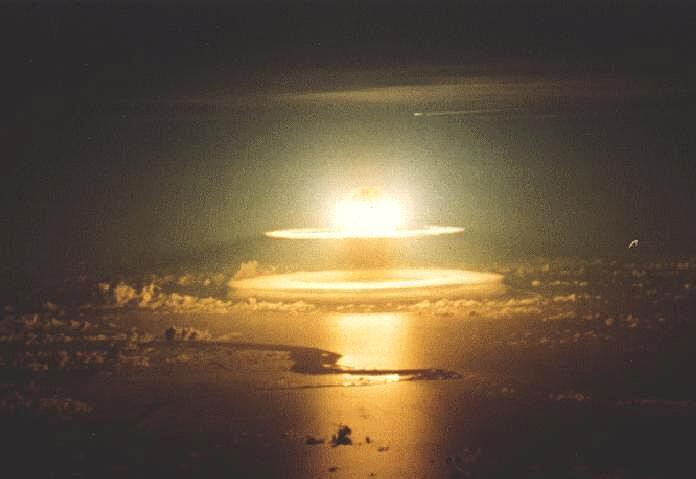Corrections toDocumentation and Diagrams of the Atomic Bomb by Arthur TsingoidasVolts:  The article refers to volts as a unit of energy. volts are not a unit of energy. One volt is actually one joule per colomb of electric charge. Voltage is often used to describe electric potential. Electron volts are a unit of energy. This unit is very small and generally used to describe atomic processes.

Gamma Radiation:  The article refers to gamma radiation as the "most powerful form of radioactivity". Alpha particles generally have greater ionizing potential than gamma radiation. What makes gamma radiation so dangerous is its ionizing potential coupled with its superior penetrating power. Alpha particles are incapable of penetrating a person's clothing and skin. Gamma radiation can go right through a person's body. Hence, gamma radiation is probably the most lethal form of nuclear radiation. But it is not necessarily the most powerful form of nuclear radiation.

Super Critical Mass:  The article states the a supercritical mass of uranium would be at least "50 kilograms". The mass of uranium required to achieve super critical mass is much less than 50 kilograms. A much smaller mass than the one quoted can be supercritical. Every U-235 nucleus that undergoes fission releases (on average) about 2 neutrons. If you expect both neutrons from each and every fission to encounter U-235 nuclei, then the mass of uranium required will need to be large. Perhaps as large as the mass you stated in the article. But what if (on average) only 1.5 neutrons per fission are absorbed by other U-235 nuclei. In other words, for every 4 neutrons that are released, 3 will find their target. The number of nuclei undergoing fission will still increase exponentially. An "uncontrolled" chain reaction will still take place. But a much smaller amount of uranium will be required. The fact that a relatively small amount of uranium is required was the key to the US developing the atomic bomb first. Heisenberg (the German leading the Nazi atomic bomb development) incorrectly assumed that all (both) neutrons need to be utilized. The mass of uranium he needed to produce an explosion was calculated as being too large (much the same as the large mass stated). It would've taken years to produce such a large amount of concentrated U-235. As a result, Heisenberg never expected anyone to build such a device.

U-238:  The article states that U-238 "reflects neutrons, rather than absorbing". U-238 also has the ability to absorb fast moving neutrons. This is how Neptunium and (eventually) Plutonium is formed.

Centrifugal force:  The article refers to centrifugal force. There is no such thing as centrifugal force. Centripetal force is the force that is associated with circular motion. Ignoring the forces due to friction, if an object is experiencing circular motion that is perpendicular to the Earth's gravitational field, then the net force on the object is towards the center. This net force is called the centripetal force. Centrifugal force is a mythical force that is, regrettably, referred to in some junior science textbooks.